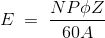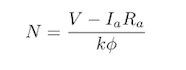# What Is DC Motor? | DC Motor’s Working Principle | Speed Control of DC MotorThe first DC motor was invented in the 18th century. Since then, this motor has been widely developed. This DC motor is significantly applied in many industries. The DC motor was developed by British explorer Sturgeon in the early 1800s and early 1832 with improvements.

He invented the early commutator type of DC motor where it has the ability to mimic machinery. But we will wonder what is the efficiency of this motor and why it is necessary to know about the speed of the DC motor. So today we will see what a DC motor is. Today we will see what is the working principle of this and how to control the speed of this DC motor.

In This Post

## What is DC Motor?The DC motor is driven by a direct current. In which it works to convert the received electrical energy into mechanical energy. This triggers a rotational change in the device thus delivering power to manage different applications across multiple domains.

DC motor speed control is one of the most useful features of a motor. By controlling the speed of the motor you can change the speed of the motor as per your requirement and get the required performance.

Speed ​​control is a mechanism applied in many cases such as controlling the movement of a robotic vehicle, the movement of a motor in a paper mill, and the movement of a motor in an elevator using a variety of DC motors.

Also Read: Difference Between AC Motor And DC Motor

## DC Motor’s Working Principle:

A simple DC motor works on the principle that when a current-carrying conductor is placed in a magnetic field, it experiences a mechanical force. In a practical DC motor, the armature is a current-carrying conductor and the field provides a magnetic field.

It produces its own magnetic flux when the current is supplied to the armature. The magnetic flux either adds to the magnetic flux due to the winding of the field in one direction or cancels the magnetic flux due to the winding of the field. The accumulation of magnetic flux in one direction exerts a force on the conductor in comparison to the other and hence it continues to rotate.

The rotating action of the conductor produces EMF according to Faraday’s law of electromagnetic induction. This opposes reason according to the EMF lens rule. This means that the supply voltage thus has a very special characteristic of adjusting its torque in case of different loads due to the EMF behind the DC motor.

### Why DC Motor Speed Control is Important?

Speed ​​control in the machine shows the effect on the speed of rotation of the motor. Where this has a direct impact on the efficiency of the machine and is of great importance for the performance and result of the operation. Each type of material has its own rotational speed when drilling and it also varies depending on the size of each drill.

The throughput rate will change from the status of the pump installation and therefore the conveyor belt needs to be synchronized with the functional speed of the device. This factor is directly or indirectly dependent on the speed of the motor. Because of this one should take into account the speed of the DC motor and observe different types of speed control methods.

DC motor speed control is done manually or using any automatic controlling tool. This seems to be contrary to the speed limit where there should be speed regulation as opposed to a natural change in speed due to a change in shaft load.

### The Principle of Speed Control:

The voltage equation of the DC motor is as follows:

`V = Eb + IaRa`

V is the given voltage while Eb is the rear EMF, Ia is the armature current, and Ra is the armature resistance.

`Eb = (PøNZ) / 60A.`

P = number of poles.

A – constant.

Z – number of conductors.

N- the speed of the motor.

By changing the value of Eb in the voltage equation, we get

```V = ((PøNZ) / 60A) + IaRa

Or, V - IaRa = (PøNZ) / 60A```

i.e., N = (PZ / 60A) (V – IaRa) /

The above equation can also be written as:

`N = K (V - IaRa) / ø, K is a constant`

This suggests three things:

• The speed of the motor is directly proportional to the voltage supply.
• The speed of the motor is inversely proportional to the armature voltage drop.
• The motor speed is inversely proportional to the flow due to field findings.

Thus, the speed of a DC motor is controlled in three ways.

• By separating the given or supply voltage.
• By changing the flux and changing the current in the field winding.
• by changing the armature voltage and changing the armature resistance.

### Multiple Techniques of DC Motor Speed Control:

There are two types of DC motors available in the market so here we will talk about the speed controlling method of both DC series and shunt motors.

Series type motor speed control is done in two ways as follows:

• Armature controlled technique.
• Field-controlled technology.

The armature control technique is classified into three types as follows:

• Armature Controlled Resistance
• Shunt armature control.
• Armature terminal voltage.

#### Armature Controlled Resistance:

This system is the most widely used in the market. The regulator in this system has a series connection to the resistance motor supply which you can see in the figure below.The power loss in controlling resistance in this motor can be ignored as this regulating technique is mostly used for the long term to reduce the visual time speed of light loading. It is a cost-effective technique for continuous torque and is mainly applied in driving cranes, trains, and other vehicles.

#### Shunted Armature Control:In this type of armature control, the rheostat will have a connection with the armature in both the series and the shunt. The voltage will change to the level that is applied to the armature and this varies by changing the rheostat of the series.

When a change in the flow of arousal occurs by changing the shunt rheostat. This method of controlling speed in a DC motor is not so expensive. Because of the significant loss of electricity in the speed regulation resistance. Speed ​​can be controlled to some extent but not above the normal level of speed.

#### Armature Terminal Voltage:

The speed of a DC series motor can also be adjusted by supplying power to the motor using different individual supply voltages. Even this approach is expensive and not widely implemented.

Field-controlled technology is further divided into two types as follows:

• Field Diverter.
• Controlling of tapped field (Tapped field control).

#### Field Diverter Technique:A diverter is used in this technique. The rate of flow across the field can be reduced by turning off some part of the motor current in the range area. The diverter resistance is low and the field flow is low.

This technique is used for more than the normal range of speeds and is applied in electric divert where the speed increases as the load decrease.

#### Controlling of Tapped Field:

Even within this system the speed increases with the reduction of the flow and this is accomplished by reducing the turn of the field from where the flow flows. Here the number of tapping in the field winding is extracted and this technique is used in electric traction.

### Speed Control of DC Shunt Motor:

The speed control of a DC shunt motor is classified into two types as follows:

• Field controlled technique.
• Armature controlled technique.

#### Field Control Method for DC Shunt Motor:

In this method, there is a variety of magnetic flux due to the field winding changing the speed of the motor.

The magnetic flux depends on the current flowing through the field winding. As such, that field can be varied by separating the current by winding. This can be achieved by using variable resistors in series with field winding resistors.

Initially when the variable resistor is kept at its minimum position. Rated current flows through the field winding due to the rated supply voltage. And as a result, the speed is kept normal. The flow through the field winding decreases when the resistance gradually increases. This in turn reduces the flow produced. Thus the speed of the motor exceeds its normal value.

#### Armature Resistance Control Method for DC Shunt Motor:

In this method, the speed of the DC motor can be controlled by controlling the resistance of the armature to control the drop in the armature. This method also uses variable resistors in series with the armature.

The armature resistance is at normal when the variable resistor reaches its minimum value and therefore the armature voltage decreases. When the resistance value gradually increases the voltage in the armature decreases and in turn leads to a decrease in the speed of the motor. This method achieves the speed of the motor below its normal range.

### DC Motor Speed Control Theory:

To achieve the speed of the DC motor we start with the equation of emf of the DC motor. The emf equation of a DC motor is exactly as we know it.

``

That’s why we’re rearranging the equation

N = 60A E / PZØ

With K = PZ / 60A, then:

N = E / kØ.

That is why with E = V – IaRa, we get the speed of the DC motor (N):

``1. What are the speed control methods of DC motor?

There are three main ways to regulate the speed of a DC series motor as follows:

• Flux control system.
• Voltage Regulation System.
• Armature resistance control system.

2. Why do we control the speed of the DC motor?

DC motors play an important role in energy conversion. This is just like any other machine which converts electrical energy into mechanical energy. In a mechanical system, the speed varies with the number of tasks so speed control is required for proper mechanical work to make the motor run smoothly.

3. Which device is used in speed control of DC motor?

In this method, the speed of the DC motor can be controlled by controlling the resistance of the armature to control the drop in the armature. This method also uses variable resistors in series with the armature.

4. What are the 3 types of DC motors?

There are three main types of DC motors available in the market: Series, Shunt, and Compound. These conditions relate to the type of connection of the field windings with respect to the armature circuit.

Like this post? Share it with your friends!